# Olympiad Test: Analytical Reasoning - 1

## 15 Questions MCQ Test Science Olympiad Class 8 | Olympiad Test: Analytical Reasoning - 1

Description
Attempt Olympiad Test: Analytical Reasoning - 1 | 15 questions in 30 minutes | Mock test for Class 8 preparation | Free important questions MCQ to study Science Olympiad Class 8 for Class 8 Exam | Download free PDF with solutions
QUESTION: 1

### In each of the following problems, find the number of triangles in the given figure :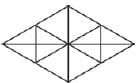Solution:

12 + 8 + 4 + 4 = 28

QUESTION: 2

### In each of the following problems, find the number of triangles in the given figure :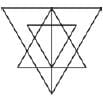Solution:

8 + 10 + 5 + 2 + 1 + 1 = 27.

QUESTION: 3

### In each of the following problems, find the number of triangles in the given figure :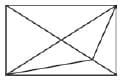Solution:

6 + 6 + 2 + 1 = 15

QUESTION: 4

In each of the following problems, find the number of triangles in the given figure :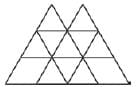Solution:

10 + 2 + 4 + 2 = 18

QUESTION: 5

In each of the following problems, find the number of triangles in the given figure :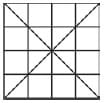Solution:

16 + 4 + 8 + 4 + 4 + 8 + 4 = 48

QUESTION: 6

In each of the following problems, find the number of triangles in the given figure :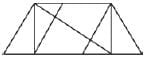Solution:

6 + 4 + 3 + 1 = 14

QUESTION: 7

In each of the following problems, find the number of triangles in the given figure :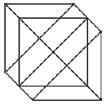Solution:
QUESTION: 8

In each of the following problems, find the number of triangles in the given figure :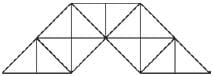Solution:
QUESTION: 9

In each of the following problems, find the number of triangles in the given figure :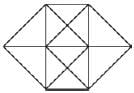Solution:
QUESTION: 10

In each of the following problems, find the number of triangles in the given figure :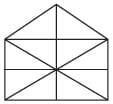Solution:
QUESTION: 11

In each of the following problems, find the number of triangles in the given figure :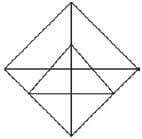Solution:
QUESTION: 12

In the following problems, count the number of squares in each figure.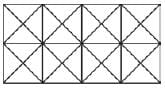Solution:

10 + 8 + 3 + 3 = 24

QUESTION: 13

In the following problems, count the number of squares in each figure.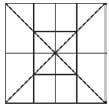Solution:

4 + 4 + 4 + 2 + 1 + 1 = 16

QUESTION: 14

In the following problems, count the number of squares in each figure.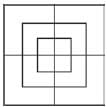Solution:
QUESTION: 15

In the following problems, count the number of squares in each figure.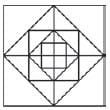Solution:Use Code STAYHOME200 and get INR 200 additional OFF Use Coupon Code